1. Motivation

Addressing many fundamental questions in biology begins with estimating the rate(s) of underlying biological processes. Although these rates are generally non-linear functions of a variety of state variables, biological research questions often focus on a putatively linear rate that must be estimated reliably, and without artefactual nonlinearies. Across many research disciplines in the biological sciences, ranging from physiology, to community ecology, to biogeochemistry, biological rates are routinely estimated from noisy, non-linear time series using simple linear regression techniques and manual truncation of nonlinear regions of the data. Further, published studies rarely provide both the raw data and estimation methods necessary to reproduce results, making it difficult to evaluate or reproduce findings from raw data to published result.

In this html_vignette, we introduce the LoLinR package, which provides tools to implement local linear regression techniques for estimating monotonic biological rates from time-series or trace data in a statistically robust and reproducible fashion. The methods are a modification of traditional Loess regression techniques built around the wrapper function rankLocReg(). We walk through several example analyses to illustrate the use and utility of the package, as well as highlight potential analytical pitfalls.

*For a full detailed description of the methods and additional example analyses, please have a look at the methods paper describing the package at http://link-to-publisher-webpage.com*.

2. Sea urchin data

We here use respiration data from the sea urchin Heliocidaris erythrogramma, common to Port Phillip Bay, Melbourne, Australia. The data are O2 consumption time-series for 4 individual urchins included in a respirometry study (Colin Olito unpublished data). For this example, the biological question of interest has to do with estimating resting metabolic rate for each individual from their O2 consumption time series. The variable include Time (min), and mL O2 data for each individual (coded by the letters A-D). After loading the data set, examination of the time series for individual ‘D’ gives a representative example of the analytical challenge presented by these data.

library(LoLinR)
data(UrchinData)
par(omi=rep(0.3, 4))
plot(D ~ time, data=UrchinData,
xlab='Time (minutes)', ylab=substitute('Volume O'~' (mL)'), type='n', axes=FALSE)
usr  <-  par('usr')
rect(usr, usr, usr, usr, col='grey90', border=NA)
whiteGrid()
box()
points(UrchinData$D ~ UrchinData$time, pch=16, col=transparentColor('dodgerblue2', 0.6), cex=1.1)
axis(1)
axis(2, las=1)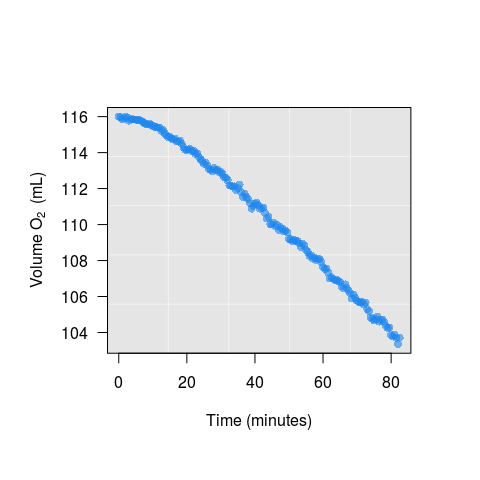The full data set is clearly non-linear. The initial 20 min of the data are messy because the animal is stressed from handling, and the (closed respiration) chamber is still equilibrating after setup. There is also a more subtle acceleration in the rate of O2 consumption towards the end of the time series as the animal exhibits a physiological response to declining O2 avaiability in the chamber. While a non-linear statistical model could conceivably be fit to these data, the question of interest requires an estimate of a simple monotonic rate. Although estimating a simple monotonic rate from these data appears to be straightforward, it is actually a non-trivial challenge. On one hand, any estimate that includes the non-linear portions of the data is not actually an estimate of the rate of interest, as it includes equipment equilibration, as well as physiological responses to stress and declining oxygen availability. On the other hand, manually truncating the data to exclude the non-linear parts is subjective, can sacrifice statistical power by excluding good data, and may be arbitrary, not reproducible, or both. Ultimately, we are interested in the region where the rate of O2 consumption is most stable, which in theory should represent the resting metabolic rate. Using , we can do this using the function.

urchinRegs  <-  rankLocReg(xall=UrchinData$time, yall=UrchinData$D, alpha=0.2,
method="eq", verbose=TRUE)  
## rankLocReg fitted 8911 local regressions

The main wrapper function rankLocReg runs all possible local regressions (on adjacent data points) with minimum window size alpha (where alpha represents the proportion of the full data). The user chooses one of three methods/metrics to quantify linearity for each local regression (z, eq or pc – default to z, see below for explanation of each method), and ranks all local regressions according the chosen metric.

Linearity Metrics Please see the methods paper here for a detailed description of the linearity metrics. Briefly, all three $$L$$ (linearity) metrics are themselves the sum of three component metrics which are calculated for each local regression. The component metrics are 1) Skewness of the standardized residuals, 2) autocorrelation of the standardized residuals, as estimated by a modified Breusch-Godfey statistic $$R^2_{BG}$$, and 3) the range of the 95% C.I. for the regression coefficient $$\beta_1$$. The main difference between the three $$L$$ metrics is how the component metrics are weighted. $$L_Z$$ represents the sum of the minimum of the $$Z$$-transformed component metrics. Thus, $$L_Z$$ implicitly weights each component metrics by their empirical variances. $$L_{eq}$$ weights each component metric equally. $$L_{\%}$$ sums the percentile-ranks of the minimum $$Z$$ scores for each metric. Ultimately, the choice of $$L$$ metrics will depend on the biology of the system being studies, the characteristics of the specific data set being analyzed, and the alpha value passed to rankLocReg. However, due to common heteroscedasticity among the component metrics, we strongly recommend the use of $$L_{eq}$$ and $$L_{\%}$$, and urge users even more strongly to carefully examine results from alternative $$L$$ metrics using the reRank function.

rankLocReg outputs an object of class rankLocReg, which includes a list of 6 items:

• nFits - the number of local regression that were fitted to the data
• allRegs - a data frame with fit and ranking data for all local regressions fit to the data
• xall - the raw data for the independent variable
• yall - the raw data for the dependent variable
• call - a summary of the call to rankLocReg
• method - the ranking method used

Several standard diagnostic functions exist for rankLocReg objects, including summary and plot.

summary(urchinRegs)
## Call:
## rankLocReg.default(xall = UrchinData$time, yall = UrchinData$D,
##     alpha = 0.2, method = "eq", verbose = TRUE)
##
## Number of fitted local regressions:
##  8911
##
## Used dataset:
##       xall            yall
##  Min.   : 0.00   Min.   :103.4
##  1st Qu.:20.62   1st Qu.:107.1
##  Median :41.25   Median :110.9
##  Mean   :41.25   Mean   :110.6
##  3rd Qu.:61.88   3rd Qu.:114.2
##  Max.   :82.50   Max.   :116.0
##
## Best  6  local regressions (L-metric ranking) fitted with method  eq
##        Lbound Rbound      alph       b0         b1     b1LoCI     b1UpCI
## x18140     95    137 0.2590361 118.2348 -0.1764844 -0.1827950 -0.1701739
## x17403     79    144 0.3975904 118.0966 -0.1745097 -0.1782557 -0.1707638
## x17389     79    130 0.3132530 117.9715 -0.1719299 -0.1775563 -0.1663034
## x18157     95    154 0.3614458 118.4510 -0.1803949 -0.1844814 -0.1763083
## x18143     95    140 0.2771084 118.3117 -0.1779081 -0.1836680 -0.1721482
## x17388     79    129 0.3072289 117.9667 -0.1718295 -0.1776828 -0.1659762
##            ciRange        skew       bgN       Lz       Leq       Lpc
## x18140 0.012621095 -0.05138762 0.8442866 4.580049 0.6620900 0.3346426
## x17403 0.007491980 -0.34618325 0.8427309 4.319476 0.6785341 0.4365765
## x17389 0.011252922 -0.34746029 0.8184386 3.231795 0.7315007 0.5183107
## x18157 0.008173071 -0.11802618 0.9024476 8.526763 0.8258289 0.3233457
## x18143 0.011519843 -0.10578746 0.8782177 7.295643 0.8459556 0.3784461
## x17388 0.011706632 -0.34919832 0.8417714 5.255905 0.8840369 0.5260913
##
## Best ranked regressions for each L-metric:
##        Metric Lbound Rbound      alph       b0         b1     b1LoCI
## x17389     Lz     79    130 0.3132530 117.9715 -0.1719299 -0.1775563
## x18140    Leq     95    137 0.2590361 118.2348 -0.1764844 -0.1827950
## x13843    Lpc     33    149 0.7048193 117.7520 -0.1686078 -0.1703633
##            b1UpCI     ciRange        skew       bgN        Lz       Leq
## x17389 -0.1663034 0.011252922 -0.34746029 0.8184386  3.231795 0.7315007
## x18140 -0.1701739 0.012621095 -0.05138762 0.8442866  4.580049 0.6620900
## x13843 -0.1668523 0.003511029  0.01103579 0.9738896 12.868442 0.8883777
##               Lpc
## x17389 0.51831070
## x18140 0.33464258
## x13843 0.05409045

A summary of a rankLocReg object provides:

• a summary of the call
• the number of local regressions that were fitted to the data
• standard summary statistics of the raw data set
• a data frame witht he top 6 ranked regressions according to the chosen $$L$$ metric
• a data frame with the number 1 ranked regression for each of the three $$L$$ metrics.

plot output for object of class rankLocReg, using the best local regression (according to method eq):

plot(urchinRegs, rank=1)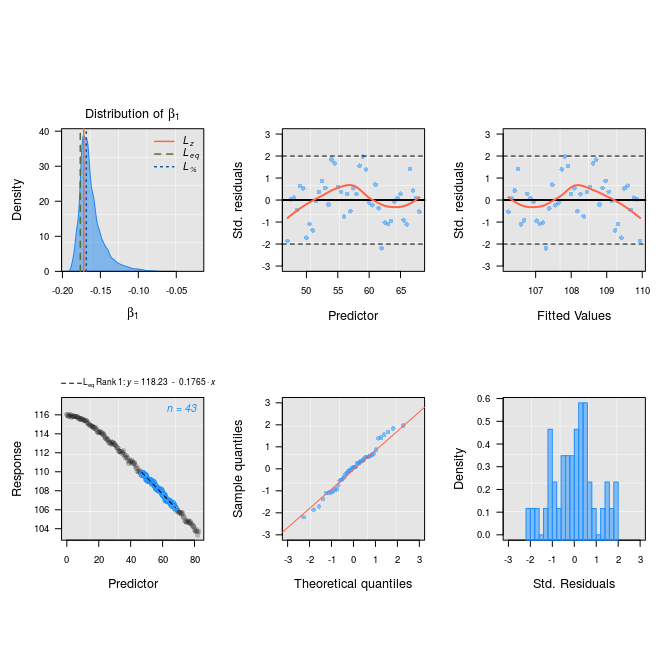Notice that the first plot provides the empirical distribution of $$\beta_1$$ for each local regression, with benchmarks for the number 1 ranked local regression for each of the three $$L$$ ranking methods. One can also inspect the best 25 local regressions following the chosen method

outputRankLocRegPlot(urchinRegs)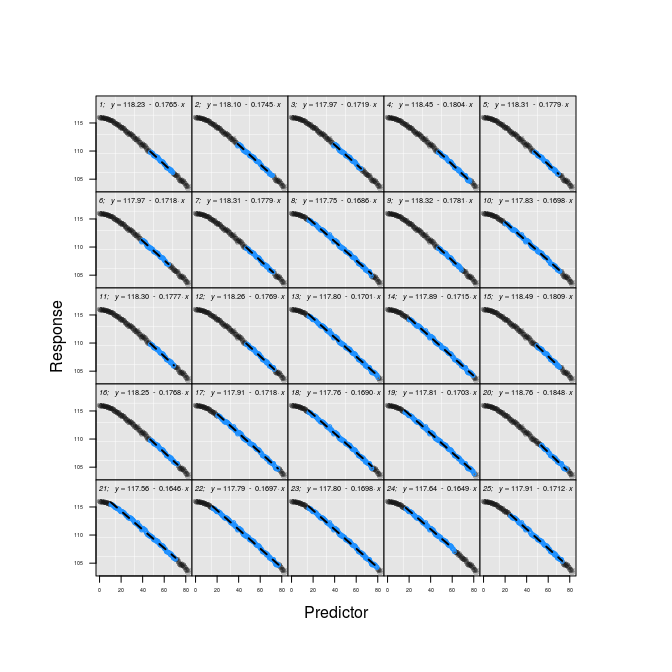3. O2 consumption in cormorant

We now show a different utility using a dataset containing O2 consumption data for a Great cormorant. This dataset represents a small example of data used for analyses described in White et al. 2011. Metabolic rate throughout the annual cycle reveals the demands of an Arctic existence in Great Cormorants. Ecology 92: 475–486. In contrast to the previous example, this data set is a time series of the rate of O$$_2$$ consumption (.$$VO_2$$ in mL O$$_2~kg^{-1}~min^{-1}$$) rather than O$$_2$$ saturation or concentration data. For this flow-through respirometry data, the question of interest is to estimate resting metabolic rate – or the region of the time series where .VO$$_2$$ was lowest, and most stable.

This data set has a relatively large number of observations. Currently, a call to LoLinR using a data set with $$N \approx 500$$ observations will take a few minutes to run, and computation time increases exponentially with larger $$N$$. So before calling rankLocReg, we thin this dataset to $$\frac{1}{3} \times N$$ using the package function thinData in order to shorten computation time. This level of thinning does not alter any important features of the full time series for this data set. However, users should always check to see how thinning changes any data set before proceeding with an analysis.

data(CormorantData)
CormorantData  <-  thinData(CormorantData, by=3)$newData1 cormRegs <- rankLocReg(xall=CormorantData$Time, yall=CormorantData$VO2.ml.min, alpha=0.2, method="eq", verbose=FALSE) As we can see, the best local regression picked up by method eq is not ideal. Instead, when inspecting the distribution of all local regression slopes (top-left plot), we see that the percentage method might be better plot(cormRegs, rank=1)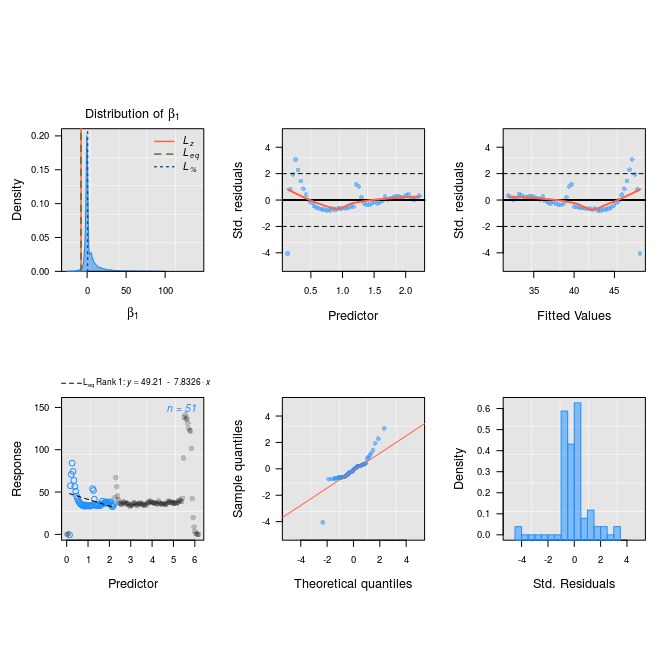So we can easily re-sort the output data.frame of local regressions using method pc cormRegs <- reRank(cormRegs, newMethod='pc') plot(cormRegs, rank=1)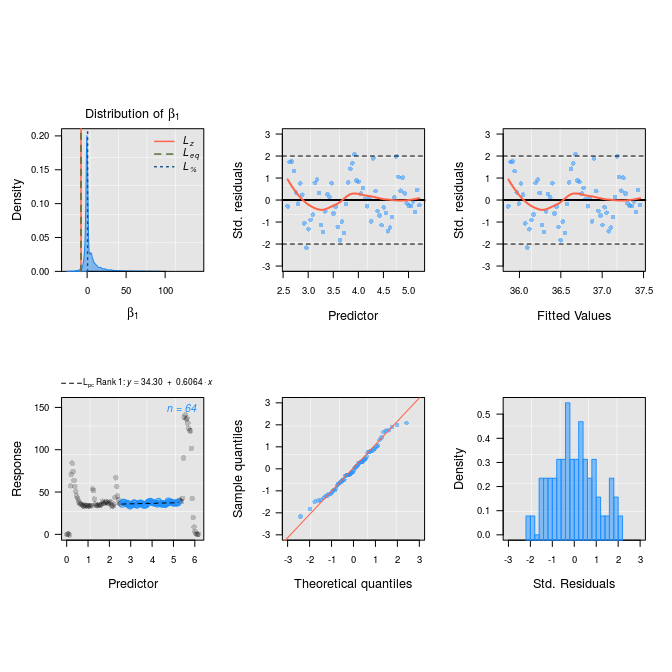Since this is a time series of .$$VO_2$$, our interest focuses on the average of the points included in the most stable region of the time series identified by rankLocReg. This can be accomplished easily by examining the Lbound and Rbound items for the best ranked regression, and subsetting the original .$$VO_2$$ data using the associated data window. summary(cormRegs)$summaryTable[1,]
##        Lbound Rbound      alph       b0        b1    b1LoCI   b1UpCI
## x15583     63    126 0.4295302 34.29595 0.6063904 0.1858365 1.026944
##          ciRange      skew       bgN       Lz       Leq        Lpc
## x15583 0.8411078 0.1770689 0.9498242 8.631553 0.9360131 0.05642792
lB  <-  summary(cormRegs)$summaryTable$Lbound
rB  <-  summary(cormRegs)$summaryTable$Rbound
mean(CormorantData\$VO2.ml.min[lB:rB])
##  36.66264

3. Facilitating objective and reproducible research

The primary value of LoLinR (in our eyes) is that it provides an objective and transparent analytic toolkit for performing what is usually a relatively simple (but often methodologically opaque) first step in many research projects in the biological sciences. Importantly, readers can reproduce any LoLinR analysis if they have

1. the raw time series data, and
2. any one of the following data/output:
• alpha OR
• LoLinR output plots OR
• LoLinR summaries OR
• full R code for the analyses being reproduced

Any of these pieces of information is easy to save and include as appendices in published studies – there is no reason that any LoLinR analysis should not be fully reproducible.